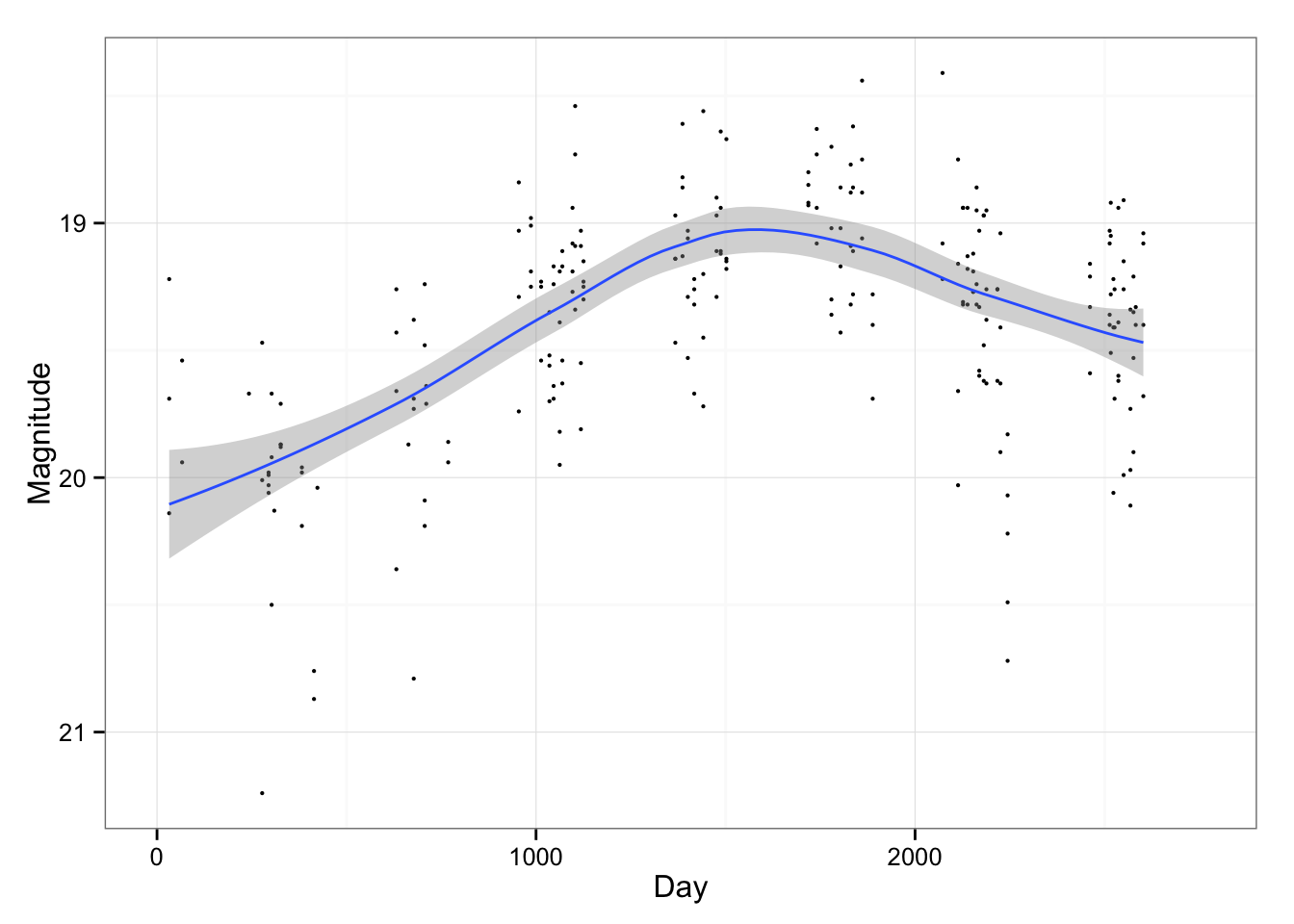# ABSTRACT

The choice of the smoothing parameter in nonparametric regression is critical to the form of the estimated curve and any inference that follows. Many methods are available that will generate a single choice for this parameter. Here we argue that the considerable uncertainty in this choice should be explicitly represented.

The construction of standard simultaneous confidence bands in nonparametric regression often requires difficult mathematical arguments. We question their practical utility, presenting several deficiencies. We propose a new kind of confidence band that reflects the uncertainty regarding the smoothness of the estimate.

# Code and Data

We use the following:

Download these if you want to reproduce the following:

# Active Galactic Nucleus Data

Read in AGN data

``agn <- read.table("agn.dat",header=TRUE)``

Compute default SCBs using lowess:

``````datarange=2764
agn\$mjd <- agn\$mjd - 53464
library(ggplot2,quietly=TRUE)
p <- ggplot(agn ,aes(x=mjd, y=mag))
p <- p+xlab("Day")+ ylab("Magnitude") + scale_y_reverse()+xlim(0,datarange)
p <- p+geom_point(size=0.75)
slow <- p + geom_smooth()
slow``````Now use smoothing splines via mgcv:

``````sspl <- p + geom_smooth(method="gam", formula = y ~ s(x, bs = "cs"))
sspl``````